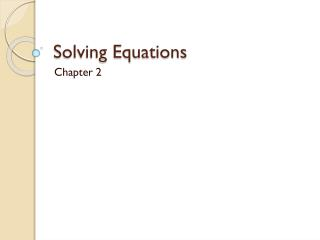DownloadDownload PresentationSolving Equations

# Solving Equations

Download Presentation## Solving Equations

- - - - - - - - - - - - - - - - - - - - - - - - - - - E N D - - - - - - - - - - - - - - - - - - - - - - - - - - -
##### Presentation Transcript

1. Solving Equations Chapter 2

2. 2.1 Solving One-Step Equations • Pg. 81 – 87 • Obj: Learn how to solve one-step equations in one variable. • Content Standard: A.CED.1 and A.REI.3

3. 2.1 Solving One-Step Equations • Equivalent equations – equations that have the same solution(s) • Isolate – get the variable with a coefficient of one alone on one side of the equation • Inverse Operation – an operation that undoes another operation

4. 2.1 Solving One-Step Equations • Method for solving one-step equations • Goal: Isolate the variable • Use the inverse operation to get the variable by itself • Addition – Subtraction • Subtraction – Addition • Multiplication – Division • Division – Multiplication • Whatever you do to one side of the equation, you must do to the other (balanced)

5. 2.2 Solving Two-Step Equations • Pg. 88 – 93 • Obj: Learn how to solve two-step equations in one variable. • Content Standards: A.REI.3, A.CED.1, and A.REI.1

6. 2.2 Solving Two-Step Equations • Method for solving two-step equations • Goal: Isolate the variable • Use the reverse of the order of operations • Addition or subtraction • Multiplication or division • Use the inverse operations • Keep the equation balanced

7. 2.3 Solving Multi-Step Equations • Pg. 94 – 100 • Obj: Learn how to solve multi-step equations in one variable. • Content Standards: A.CED.1, A.REI.1, and A.REI.3

8. 2.4 Solving Equations With Variables on Both Sides • Pg. 102- 108 • Obj: Learn how to solve equations with variables on both sides and identify equations that are identities or have no solution. • Content Standards: A.CED.1, A.REI.1, and A.REI.3

9. 2.4 Solving Equations With Variables on Both Sides • Identity – an equation that is true for every possible value of the variable

10. 2.4 Solving Equations With Variables on Both Sides • Solving Equations • Use the Distributive Property to remove grouping symbols • Combine like terms on each side of the equation • Get the variable terms on one side of the equation • Use the inverse operations to solve for the variable • Check you solution in the original equation

11. 2.5 Literal Equations and Formulas • Pg. 109 – 114 • Obj: Learn how to rewrite and use literal equations and formulas. • Content Standards: A.CED.4, N.Q.1, A.CED.1, A.REI.1, A.REI.3

12. 2.5 Literal Equations and Formulas • Literal equation - an equation that involves two or more variables • Formula – an equation that states a relationship among quantities

13. 2.5 Literal Equations and Formulas

14. 2.6 Ratios, Rates, and Conversions • Pg.116 – 121 • Obj: Learn how to find ratios and rates and convert units and rates. • Content Standards: N.Q.1 and N.Q.2

15. 2.6 Ratios, Rates, and Conversions • Ratio – compares two numbers by division • Rate – a ratio that compares quantities measured in different units • Unit Rate – a rate with a denominator of 1 • Conversion Factor – a ratio of two equivalent measures in different units • Unit Analysis – using the units in calculations to help determine the units for the answers

16. 2.7 Solving Proportions • Pg. 124 – 129 • Obj: Learn how to solve and apply proportions. • Content Standards: A.REI.3, N.Q.1, and A.CED.1

17. 2.7 Solving Proportions • Proportion – an equation that states that two ratios are equal • Cross Products Property

18. 2.8 Proportions and Similar Figures • Pg. 130 – 136 • Obj: Learn how to find missing lengths in similar figures and use similar figures when measuring indirectly. • Content Standards: A.CED.1 and A.REI.3

19. 2.8 Proportions and Similar Figures • Similar Figures – have the same shape but necessarily the same size • Scale Drawing – a drawing that is similar to an actual object or place • Scale – the ratio of any length on the drawing to the actual length • Scale Model – a 3D model that is similar to the 3D object

20. 2.9 Percents • Pg. 137 – 143 • Obj: Learn how to solve percent problems using proportions and the percent equation. • Content Standard: Prepares for N.Q.3

21. 2.9 Percents • The Percent Proportion

22. 2.9 Percents • Simple Interest Formula • I = Prt • I = interest • P = Principal • r = rate (as a decimal) • t = time (in years)

23. 2.10 Change Expressed as a Percent • Pg. 144 – 150 • Obj: Learn how to find the percent of change and to find the relative error in linear and nonlinear measurements. • Content Standard: N.Q.3

24. 2.10 Change Expressed as a Percent • Percent of Change – expresses an amount of change as a percent of an original amount • Percent Increase – if a new amount is greater than the original amount • Percent Decrease – if a new amount is less than the original amount

25. 2.10 Change Expressed as a Percent • Percent of Change

26. 2.10 Change Expressed as a Percent • Relative Error – the ration of the absolute value of the difference of a measured value and an actual value compared to the actual value • Percent Error – when relative error is expressed as a percent

27. 2.10 Change Expressed as a Percent • Relative Error